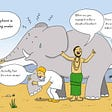# Learning Hidden Markov Models with simple examples and Matlab [part 2]

In the previous part, we covered the first task of the presented example. In this part, I’ll present the solution to the second task of the problem and try to explain the intuition behind it.

To have a fresh memory of what the problem was, here it is once again:

Consider an HMM with the following properties:

• Hidden state set Q = {1,2,3}
• Observable state set V = {a, b}
• Parameter λ = (π, A, B)

where matrices A (transition matrix), B (emission matrix), π (initial distribution) are defined as follows:

We concern ourselves with a small time step of T = 3 with the observed sequence being O = (a, b, a).

Given this information, we’ll need to:

1. Use the Forward algorithm to calculate P(O|λ). So, what is the probability that such a sequence “a → b → a” actually occurs? → DONE
2. Find the optimal hidden state sequence I* = (i₁*, i₂*, i₃*). Meaning, which are the 3 hidden states with the highest possibility of being behind the sequence “a → b → a”?

Solution to part 2:

To find out which is the most probable hidden state at each time step, it’s necessary to find the maximum product over the following properties:

1. Probability of having the current observed state given the current hidden state
2. Probability of having the current hidden state given the last hidden state
3. Probability of having the last hidden state

The important aspects here are:

• We have evidence of the observed state at each time step, that is given in the task as O = (a, b, a)!
• We don’t have to look further back past the last hidden state, because the last hidden state “blocks” the influence of any previous evidence from the Markov chain.

If you’re not certain about the second aspect I mentioned, be sure to check out the concept of conditional independence (I found this article on Medium you can check, but feel free to use any resource that helps you understand).

Once this is established, we can give the mathematical definition of what needs to be calculated at each step:

Then, it’s a matter of calculating the most probable hidden state for each time step.

First time step:

Here, you can see that the highest probability is that of hidden state 3.

Second time step:

I decided to write out the calculations as detailed as possible so that they’re to follow. Essentially, the probabilities of each possible hidden state are calculated taking into account the probabilities of the previous hidden states. From this, you can see the most probable hidden state at the second time step is 2.

Third time step:

Performing the same calculations as in the second time step, the result is the probabilities for the hidden states at the third time step.

Once we have the three vectors, we just need to take the argmax-function at each time step and this gives us the optimal hidden state sequence:

• I* = (i₁*, i₂*, i₃*) = (3, 2, 3)

So, to put it in words, if you look at the Markov chain from the beginning and observe “a → b → a” during the first 3 time steps, the hidden states with the highest probability to produce this sequence are “3 → 2 → 3”.

Hopefully, this article was concise and straightforward, but most of all, I hope it was helpful. I’ll see that the last article which discusses an HMM problem solution using Matlab and its great features is online in the upcoming days as well.

Comments, corrections, and other remarks are always appreciated.

[Part 1]

Sources:
Thumbnail picture: https://devopedia.org/images/article/214/3976.1567701977.png

--

--

--

Electrical engineer turned software (or I’d like to think so). Trying to learn + share new topics. Past internship blog posts: https://hack.bg/author/aleksandar

Love podcasts or audiobooks? Learn on the go with our new app.

## Machine learning ?## Object Detection — YOLO(You Look Only Once)## Day-49 Model Selection-2 (Grid Search)## Reinforcement Learning: Multi-armed Bandits## Neural Machine Translation — with Attention and Tensorflow 2.0## Step by Step Machine Translation using Transformer and Multi Head Attention## Ensemble Methods ≠ Best Results: an example and the reasons behind itElectrical engineer turned software (or I’d like to think so). Trying to learn + share new topics. Past internship blog posts: https://hack.bg/author/aleksandar

## Fixed Feature Extractor as the Transfer Learning Method for Image Classification Using MobileNet## Norm Exchange: Feature-Level Data Augmentation for Enhancing Neural Networks [CVPR 2021]## Deep Neural Network Overview Part 2## Technical debt in Machine Learning